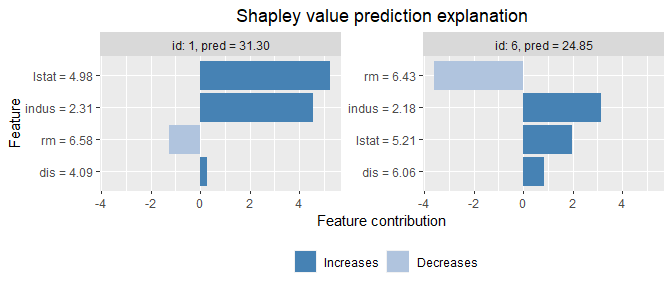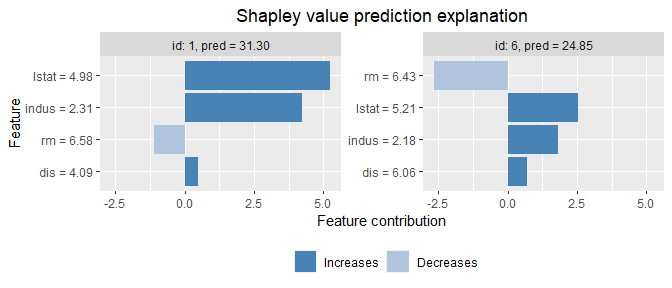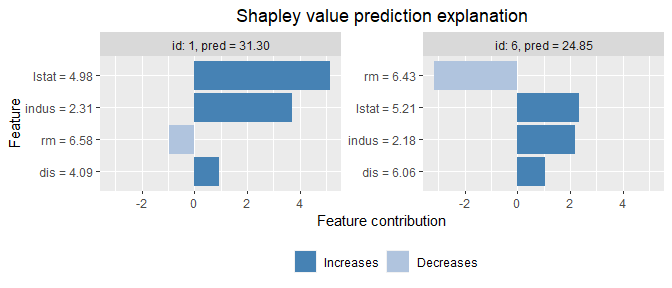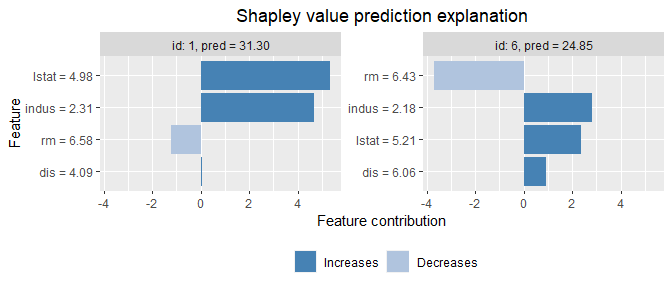# shapr: Explaining individual machine learning predictions with Shapley values

Introduction

Overview of Package

The Kernel SHAP Method

Examples

Comparison to Lundberg & Lee’s implementation

# Introduction

The shapr package implements an extended version of the Kernel SHAP method for approximating Shapley values (Lundberg and Lee (2017)), in which dependence between the features is taken into account (Aas, Jullum, and Løland (2019)). Estimation of Shapley values is of interest when attempting to explain complex machine learning models. Of existing work on interpreting individual predictions, Shapley values is regarded to be the only model-agnostic explanation method with a solid theoretical foundation (Lundberg and Lee (2017)). Kernel SHAP is a computationally efficient approximation to Shapley values in higher dimensions, but it assumes independent features. Aas, Jullum, and Løland (2019) extend the Kernel SHAP method to handle dependent features, resulting in more accurate approximations to the true Shapley values. See the paper (Aas, Jullum, and Løland (2019)) for further details.

# Overview of Package

## Functions

Here is an overview of the main functions. You can read their documentation and see examples with ?function_name.

Main functions in the shapr package.
Function Name Description
shapr Get Shapley weights for test data.
explain Computes kernel SHAP values for test data.
plot.shapr Plots the individual prediction explanations. Uses facet_wrap of ggplot.

# The Kernel SHAP Method

Assume a predictive model $$f(\boldsymbol{x})$$ for a response value $$y$$ with features $$\boldsymbol{x}\in \mathbb{R}^M$$, trained on a training set, and that we want to explain the predictions for new sets of data. This may be done using ideas from cooperative game theory, letting a single prediction take the place of the game being played and the features the place of the players. Letting $$N$$ denote the set of all $$M$$ players, and $$S \subseteq N$$ be a subset of $$|S|$$ players, the “contribution” function $$v(S)$$ describes the total expected sum of payoffs the members of $$S$$ can obtain by cooperation. The Shapley value (Shapley (1953)) is one way to distribute the total gains to the players, assuming that they all collaborate. The amount that player $$i$$ gets is then

$\phi_i(v) = \phi_i = \sum_{S \subseteq N \setminus\{i\}} \frac{|S| ! (M-| S| - 1)!}{M!}(v(S\cup \{i\})-v(S)),$

that is, a weighted mean over all subsets $$S$$ of players not containing player $$i$$. Lundberg and Lee (2017) define the contribution function for a certain subset $$S$$ of these features $$\boldsymbol{x}_S$$ as $$v(S) = \mbox{E}[f(\boldsymbol{x})|\boldsymbol{x}_S]$$, the expected output of the predictive model conditional on the feature values of the subset. Lundberg and Lee (2017) names this type of Shapley values SHAP (SHapley Additive exPlanation) values. Since the conditional expectations can be written as

$\begin{equation} \label{eq:CondExp} E[f(\boldsymbol{x})|\boldsymbol{x}_s=\boldsymbol{x}_S^*] = E[f(\boldsymbol{x}_{\bar{S}},\boldsymbol{x}_S)|\boldsymbol{x}_S=\boldsymbol{x}_S^*] = \int f(\boldsymbol{x}_{\bar{S}},\boldsymbol{x}_S^*)\,p(\boldsymbol{x}_{\bar{S}}|\boldsymbol{x}_S=\boldsymbol{x}_S^*)d\boldsymbol{x}_{\bar{S}}, \end{equation}$

the conditional distributions $$p(\boldsymbol{x}_{\bar{S}}|\boldsymbol{x}_S=\boldsymbol{x}_S^*)$$ are needed to compute the contributions. The Kernel SHAP method of Lundberg and Lee (2017) assumes feature independence, so that $$p(\boldsymbol{x}_{\bar{S}}|\boldsymbol{x}_S=\boldsymbol{x}_S^*)=p(\boldsymbol{x}_{\bar{S}})$$. If samples $$\boldsymbol{x}_{\bar{S}}^{k}, k=1,\ldots,K$$, from $$p(\boldsymbol{x}_{\bar{S}}|\boldsymbol{x}_S=\boldsymbol{x}_S^*)$$ are available, the conditional expectation in above can be approximated by

$\begin{equation} v_{\text{KerSHAP}}(S) = \frac{1}{K}\sum_{k=1}^K f(\boldsymbol{x}_{\bar{S}}^{k},\boldsymbol{x}_S^*). \end{equation}$

In Kernel SHAP, $$\boldsymbol{x}_{\bar{S}}^{k}, k=1,\ldots,K$$ are sampled from the $$\bar{S}$$-part of the training data, independently of $$\boldsymbol{x}_{S}$$. This is motivated by using the training set as the empirical distribution of $$\boldsymbol{x}_{\bar{S}}$$, and assuming that $$\boldsymbol{x}_{\bar{S}}$$ is independent of $$\boldsymbol{x}_S=\boldsymbol{x}_S^*$$. Due to the independence assumption, if the features in a given model are highly dependent, the Kernel SHAP method may give a completely wrong answer. This can be avoided by estimating the conditional distribution $$p(\boldsymbol{x}_{\bar{S}}|\boldsymbol{x}_S=\boldsymbol{x}_S^*)$$ directly and generating samples from this distribution. With this small change, the contributions and Shapley values may then be approximated as in the ordinary Kernel SHAP framework. Aas, Jullum, and Løland (2019) propose three different approaches for estimating the conditional probabilities. The methods may also be combined, such that e.g. one method is used when conditioning on a small number of features, while another method is used otherwise.

## Multivariate Gaussian Distribution Approach

The first approach arises from the assumption that the feature vector $$\boldsymbol{x}$$ stems from a multivariate Gaussian distribution with some mean vector $$\boldsymbol{\mu}$$ and covariance matrix $$\boldsymbol{\Sigma}$$. Under this assumption, the conditional distribution $$p(\boldsymbol{x}_{\bar{\mathcal{S}}} |\boldsymbol{x}_{\mathcal{S}}=\boldsymbol{x}_{\mathcal{S}}^*)$$ is also multivariate Gaussian
$$\text{N}_{|\bar{\mathcal{S}}|}(\boldsymbol{\mu}_{\bar{\mathcal{S}}|\mathcal{S}},\boldsymbol{\Sigma}_{\bar{\mathcal{S}}|\mathcal{S}})$$, with analytical expressions for the conditional mean vector $$\boldsymbol{\mu}_{\bar{\mathcal{S}}|\mathcal{S}}$$ and covariance matrix $$\boldsymbol{\Sigma}_{\bar{\mathcal{S}}|\mathcal{S}}$$, see Aas, Jullum, and Løland (2019) for details. Hence, instead of sampling from the marginal empirical distribution of $$\boldsymbol{x}_{\bar{\mathcal{S}}}$$ approximated by the training data, we can sample from the Gaussian conditional distribution, which is fitted using the training data. Using the resulting samples $$\boldsymbol{x}_{\bar{\mathcal{S}}}^k, k=1,\ldots,K$$, the conditional expectations be approximated as in the Kernel SHAP.

## Gaussian Copula Approach

If the features are far from multivariate Gaussian, an alternative approach is to instead represent the marginals by their empirical distributions, and model the dependence structure by a Gaussian copula. Assuming a Gaussian copula, we may convert the marginals of the training data to Gaussian features using their empirical distributions, and then fit a multivariate Gaussian distribution to these.

To produce samples from the conditional distribution $$p(\boldsymbol{x}_{\bar{\mathcal{S}}} |\boldsymbol{x}_{\mathcal{S}}=\boldsymbol{x}_{\mathcal{S}}^*)$$, we convert the marginals of $$\boldsymbol{x}_{\mathcal{S}}$$ to Gaussians, sample from the conditional Gaussian distribution as above, and convert the marginals of the samples back to the original distribution. Those samples are then used to approximate the sample from the resulting multivariate Gaussian conditional distribution. While other copulas may be used, the Gaussian copula has the benefit that we may use the analytical expressions for the conditionals $$\boldsymbol{\mu}_{\bar{\mathcal{S}}|\mathcal{S}}$$ and $$\boldsymbol{\Sigma}_{\bar{\mathcal{S}}|\mathcal{S}}$$. Finally, we may convert the marginals back to their original distribution, and use the resulting samples to approximate the conditional expectations as in the Kernel SHAP.

## Empirical Conditional Distribution Approach

If both the dependence structure and the marginal distributions of $$\boldsymbol{x}$$ are very far from the Gaussian, neither of the two aforementioned methods will work very well. Few methods exists for the non-parametric estimation of conditional densities, and the classic kernel estimator (Rosenblatt (1956)) for non-parametric density estimation suffers greatly from the curse of dimensionality and does not provide a way to generate samples from the estimated distribution. For such situations, Aas, Jullum, and Løland (2019) propose an empirical conditional approach to sample approximately from $$p(\boldsymbol{x}_{\bar{\mathcal{S}}}|\boldsymbol{x}_{\mathcal{S}}^*)$$. The idea is to compute weights $$w_{\mathcal{S}}(\boldsymbol{x}^*,\boldsymbol{x}^i),\ i=1,...,n_{\text{train}}$$ for all training instances based on their Mahalanobis distances (in the $$S$$ subset only) to the instance $$\boldsymbol{x}^*$$ to be explained. Instead of sampling from this weighted (conditional) empirical distribution, Aas, Jullum, and Løland (2019) suggests a more efficient variant, using only the $$K$$ instances with the largest weights:

$v_{\text{condKerSHAP}}(\mathcal{S}) = \frac{\sum_{k=1}^K w_{\mathcal{S}}(\boldsymbol{x}^*, \boldsymbol{x}^{[k]}) f(\boldsymbol{x}_{\bar{\mathcal{S}}}^{[k]}, \boldsymbol{x}_{\mathcal{S}}^*)}{\sum_{k=1}^K w_{\mathcal{S}}(\boldsymbol{x}^*,\boldsymbol{x}^{[k]})},$

The number of samples $$K$$ to be used in the approximate prediction can for instance be chosen such that the $$K$$ largest weights accounts for a fraction $$\eta$$, for example $$0.9$$, of the total weight. If $$K$$ exceeds a certain limit, for instance $$5,000$$, it might be set to that limit. A bandwidth parameter $$\sigma$$ used to scale the weights, must also be specified. This choice may be viewed as a bias-variance trade-off. A small $$\sigma$$ puts most of the weight to a few of the closest training observations and thereby gives low bias, but high variance. When $$\sigma \rightarrow \infty$$, this method converges to the original Kernel SHAP assuming feature independence. Typically, when the features are highly dependent, a small $$\sigma$$ is typically needed such that the bias does not dominate. Aas, Jullum, and Løland (2019) show that a proper criterion for selecting $$\sigma$$ is a small-sample-size corrected version of the AIC known as AICc. As calculation of it is computationally intensive, an approximate version of the selection criterion is also suggested. Details on this is found in Aas, Jullum, and Løland (2019).

# Examples

shapr supports computation of Shapley values with any predictive model which takes a set of numeric features and produces a numeric outcome.

The following example shows how a simple xgboost model is trained using the Boston Housing Data, and how shapr can be used to explain the individual predictions. Note that empirical conditional distribution approach is the default (i.e. approach = "empirical"), and that the Gaussian and Gaussian copula approaches can be used instead by setting the argument approach to either "gaussian" or "copula".

library(xgboost)
library(shapr)

data("Boston", package = "MASS")

x_var <- c("lstat", "rm", "dis", "indus")
y_var <- "medv"

x_train <- as.matrix(Boston[-1:-6, x_var])
y_train <- Boston[-1:-6, y_var]
x_test <- as.matrix(Boston[1:6, x_var])

# Fitting a basic xgboost model to the training data
model <- xgboost(
data = x_train,
label = y_train,
nround = 20,
verbose = FALSE
)

# Prepare the data for explanation
explainer <- shapr(x_train, model)

# Specifying the phi_0, i.e. the expected prediction without any features
p <- mean(y_train)

# Computing the actual Shapley values with kernelSHAP accounting for feature dependence using
# the empirical (conditional) distribution approach with bandwidth parameter sigma = 0.1 (default)
explanation <- explain(
x_test,
approach = "empirical",
explainer = explainer,
prediction_zero = p
)

# Printing the Shapley values for the test data.
print(explanation$dt) #> none lstat rm dis indus #> 1: 22.446 5.2632030 -1.2526613 0.2920444 4.5528644 #> 2: 22.446 0.1671903 -0.7088405 0.9689007 0.3786871 #> 3: 22.446 5.9888016 5.5450861 0.5660136 -1.4304350 #> 4: 22.446 8.2142203 0.7507569 0.1893368 1.8298305 #> 5: 22.446 0.5059890 5.6875106 0.8432240 2.2471152 #> 6: 22.446 1.9929674 -3.6001959 0.8601984 3.1510531 # Plot the resulting explanations for observations 1 and 6 plot(explanation, plot_phi0 = FALSE, index_x_test = c(1, 6))The Gaussian approach is used as follows: # Use the Gaussian approach explanation_gaussian <- explain( x_test, approach = "gaussian", explainer = explainer, prediction_zero = p ) # Plot the resulting explanations for observations 1 and 6 plot(explanation_gaussian, plot_phi0 = FALSE, index_x_test = c(1, 6))The Gaussian copula approach is used as follows: # Use the Gaussian copula approach explanation_copula <- explain( x_test, approach = "copula", explainer = explainer, prediction_zero = p ) # Plot the resulting explanations for observations 1 and 6, excluding # the no-covariate effect plot(explanation_copula, plot_phi0 = FALSE, index_x_test = c(1, 6))## Main arguments in shapr When using shapr, the default behavior is to use all feature combinations in the Shapley formula. Kernel SHAP’s sampling based approach may be used by specifying n_combinations, which is the number of feature combinations to sample. If not specified, the exact method is used. The computation time grows approximately exponentially with the number of samples. The training data and the model whose predictions we wish to explain must be provided through the arguments x and model. Note that x must be a data.frame or a matrix, and all elements must be finite numerical values. Currently we do not support categorical features or missing values. ## Main arguments in explain The test data given by x, whose predicted values we wish to explain, must be provided. Note that x must be a data.frame or a matrix, where all elements are finite numerical values. One must also provide the object returned by shapr through the argument explainer. The default approach when computing the Shapley values is the empirical approach (i.e. approach = "empirical"). If you’d like to use a different approach you’ll need to set approach equal to either copula or gaussian, or a vector of them, with length equal to the number of features. If a vector, a combined approach is used, and element i indicates the approach to use when conditioning on i variables. For more details see Combined approach below. When computing the kernel SHAP values by explain, the maximum number of samples to use in the Monte Carlo integration for every conditional expectation is controlled by the argument n_samples (default equals 1000). The computation time grows approximately linear with this number. You will also need to pass a numeric value for the argument prediction_zero, which represents the prediction value when not conditioning on any features. We recommend setting this equal to the mean of the response, but other values, like the mean prediction of a large test data set is also a possibility. If the empirical method is used, specific settings for that approach, like a vector of fixed $$\sigma$$ values can be specified through the argument sigma_vec. See ?explain for more information. If approach = "gaussian", you may specify the mean vector and covariance matrix of the data generating distribution by the arguments mu and cov_mat. If not specified, they are estimated from the training data. # Advanced usage ## Combined approach In addition to letting the user select one of the three aforementioned approaches for estimating the conditional distribution of the data (i.e. approach equals either "gaussian", "copula" or "empirical"), the package allows the user to combine the three approaches. To simplify the usage, the flexibility is restricted such that the same approach is used when conditioning on the same number of features. This is also in line Aas, Jullum, and Løland (2019, Section 3.4). This can be done by setting approach equal to a character vector, where the length of the vector is equal to the number of features in the model. Consider a situation where you have trained a model that consists of 10 features, and you would like to use the "empirical" approach when you condition on 1-3 features, the "copula" approach when you condition on 4-5 features, and the "gaussian" approach when conditioning on 6 or more features. This can be applied by simply passing approach = c(rep("empirical", 3), rep("copula", 2), rep("gaussian", 5)), i.e. approach[i] determines which method to use when conditioning on i features. The code below exemplifies this approach for a case where there are four features, using "empirical", "copula" and "gaussian" when conditioning on respectively 1, 2 and 3-4 features. Note that it does not matter what method that is specified when conditioning on all features, as that equals the actual prediction regardless of the specified approach. # Use the combined approach explanation_combined <- explain( x_test, approach = c("empirical", "copula", "gaussian", "gaussian"), explainer = explainer, prediction_zero = p ) # Plot the resulting explanations for observations 1 and 6, excluding # the no-covariate effect plot(explanation_combined, plot_phi0 = FALSE, index_x_test = c(1, 6))## Explain custom models shapr currently supports explanation of predictions from models fitted with the following functions: • stats::lm • stats::glm • ranger::ranger • mgcv::gam • xgboost::xgboost/xgboost::xgb.train Any continuous response regression model or binary classification model of these model classes, can be explained with the package directly as exemplified above. Moreover, essentially any feature dependent prediction model can be explained by the package by specifying two simple additional functions to the class your model belongs to. The first class function is model_type, taking the model object as input, and outputting a character indicating whether the model is a (continuous response) "regression" model, or a (binary) "classification" model. The second function is predict_model, taking the model and data (as a matrix or data.frame) as input and outputting the corresponding prediction as a numeric vector. Once these two class functions are created, one can explain predictions from this model class as before. Below we exemplify this process by explaining a gbm model using the gbm package, fitted to the same Boston data set as used above. library(gbm) #> Loaded gbm 2.1.8 form <- as.formula(paste0(y_var, "~", paste0(x_var, collapse = "+"))) xy_train <- data.frame(x_train, medv = y_train) # Fitting a gbm model set.seed(825) model <- gbm::gbm( form, data = xy_train, distribution = "gaussian" ) # Create custom function of model_type for gbm model_type.gbm <- function(x) { ifelse( x$distribution$name %in% c("bernoulli", "adaboost"), "classification", "regression" ) } # Create custom function of predict_model for gbm predict_model.gbm <- function(x, newdata) { if (!requireNamespace('gbm', quietly = TRUE)) { stop('The gbm package is required for predicting train models') } model_type <- model_type(x) if (model_type == "classification") { predict(x, as.data.frame(newdata), type = "response", n.trees = x$n.trees)
} else {

predict(x, as.data.frame(newdata), n.trees = x\$n.trees)
}
}

# Prepare the data for explanation
set.seed(123)
explainer <- shapr(xy_train, model, feature_labels = x_var)

# Spedifying the phi_0, i.e. the expected prediction without any features
p0 <- mean(xy_train[, y_var])

# Computing the actual Shapley values with kernelSHAP accounting for feature dependence using
# the empirical (conditional) distribution approach with
# bandwidth parameter sigma = 0.1 (default)
explanation <- explain(
x_test,
explainer,
approach = "empirical",
prediction_zero = p0
)

# Plot the resulting explanations for observations 1 and 6, excluding
# the no-covariate effect.
plot(explanation_combined, plot_phi0 = FALSE, index_x_test = c(1, 6))Note that this explains a different (but similar) model than the above xgboost model!

# Comparison to Lundberg & Lee’s implementation

As mentioned above, the original (independence assuming) Kernel SHAP implementation can be approximated by setting a large $$\sigma$$ value using our empirical approach. If we specify that the distances to all training observations should be used (i.e. setting approach = "empirical" and w_threshold = 1 when using explain, we can approximate the original method arbitrarily well by increasing $$\sigma$$. For completeness of the shapr, we have also implemented a version of the original method, which samples training observations independently with respect to their distances to test observations (i.e. without the large-$$\sigma$$ approximation). This method is available by using approach = "empirical" and type = "independence" in explain.

We have compared the results using these two variants with the original implementation of Lundberg and Lee (2017), available through the Python library shap. As above, we used the Boston housing data, trained via xgboost. We specify that all training observations should be used when explaining all of the 6 test observations. To run the individual explanation method in the shap Python library we use the reticulate R-package, allowing Python code to run within R. As this requires installation of Python package, the comparison code and results is not included in this vignette, but can be found here. As indicated by the (commented out) results in the file above both methods in our R-package give (up to numerical approximation error) identical results to the original implementation in the Python shap library.